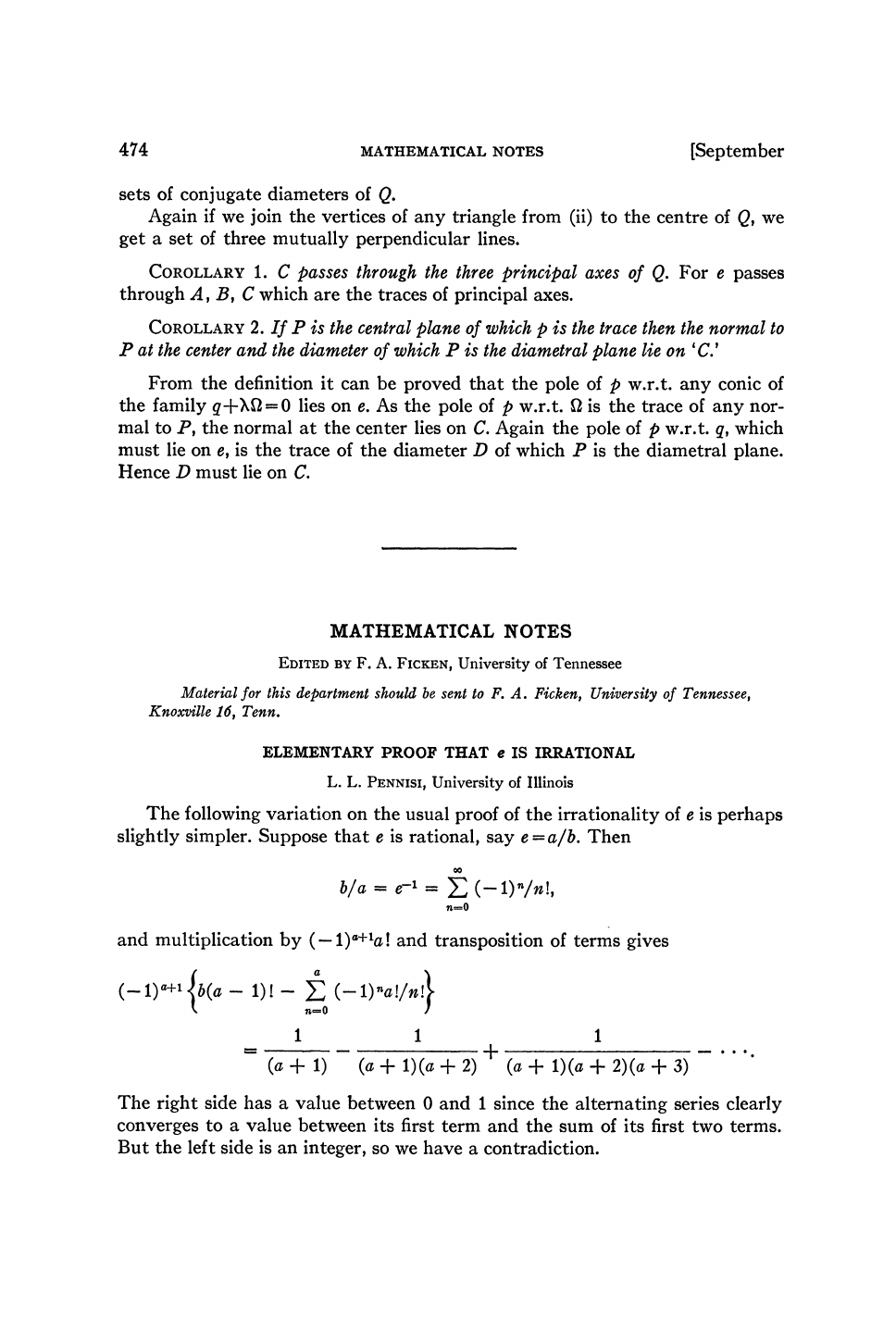The first references to the constant "e" were published in 1618 in ...
If we multiply both sides by $(-1)^{a+1}a!$ we end up with $$(...The first references to the constant "e" were published in 1618 in the table of an appendix of a work on logarithms by John Napier, which didn't contain the constant itself, but simply a list of logarithms calculated from the constant. The discovery of the constant itself is credited to Jacob Bernoulli, who in 1683 looked at the problem of compound interest and, in examining continuous compound interest, he tried to find the limit of (1 + 1/n)^n as n tends to infinity. He used the binomial theorem to show that the limit had to lie between 2 and 3 so we could consider this to be the first approximation found to e. ![](https://upload.wikimedia.org/wikipedia/commons/thumb/1/19/Jakob_Bernoulli.jpg/200px-Jakob_Bernoulli.jpg) The first proof that e is irrational was written by Euler in 1737. Jonathan Sondow proved that e was irrational using only [geometric arguments](http://fermatslibrary.com/s/a-geometric-proof-that-e-is-irrational). You are missing a (-1)^{a+1} on the RHS in the 3rd equation, i.e. for S If we multiply both sides by (-1)^{a+1}a! we end up with$$ (-1)^{a+1}\frac{a!}{a}b=(-1)^{a+1}a!\sum_{n=0}^{\infty} \frac{(-1)^{n}}{n!} $$Now, if we divide the infinite series in 2 : one from n=0 to n=a and another one from n=a+1 to n=\infty we get$$ (-1)^{a+1}(a-1)!b=(-1)^{a+1}a!\left[\sum_{n=0}^{a} \frac{(-1)^{n}}{n!}+\sum_{n=a+1}^{\infty} \frac{(-1)^{n}}{n!} \right] \\ (-1)^{a+1}\left[(a-1)!b + \sum_{n=0}^{a} \frac{(-1)^{n}a!}{n!} \right] = \sum_{n=a+1}^{\infty} \frac{(-1)^{n}a!}{n!} $$On the left side of the equality above (a-1)!b is an integer and \sum_{n=0}^{a} \frac{(-1)^{n}a!}{n!} is also going to be an integer since n \leq a, which means the left side result is an integer. Looking now at right side we have$$ S=\sum_{n=a+1}^{\infty} \frac{(-1)^{n}a!}{n!} = \\ \overbrace{\frac{1}{(a+1)}}^{s_0}- \overbrace{\frac{1}{(a+1)(a+2)}}^{s_1}+\overbrace{\frac{1}{(a+1)(a+2)(a+3)}}^{s_2}- ... $$s_n is a convergent alternating series since \lim_{n \rightarrow \infty} s_{n} = 0 and s_n is a decreasing sequence (you can read more about the Alternating Series Convergence Test [here](http://tutorial.math.lamar.edu/Classes/CalcII/AlternatingSeries.aspx)). In particular, we are going to prove that$$ 0<S<1 $$To do so, we notice that every odd partial sum \bar S_{odd} (a sum with an odd number of terms) like$$ s_{0}-s_{1}+s_{2}- s_{3}+s_{4} = s_{0}-(s_{1}-s_{2})- (s_{3}-s_{4}) $$Since s_n is a decreasing sequence, s_1>s_2 and s_3>s_4$$ s_{0}-\overbrace{(s_{1}-s_{2})}^{>0}- \overbrace{(s_{3}-s_{4})}^{>0}<s_{0} \\ \bar S_{odd} < s_0 $$At the same time for an even partial sum \bar S_{even} (a sum with an even number of terms)$$ s_{0}-s_{1}+s_{2}- s_{3}+s_{4}- s_{5} = \\ s_{0}-s_{1}+\overbrace{(s_{2}- s_{3})}^{>0}+\overbrace{(s_{4}- s_{5})}^{>0}>s_{0}-s_{1} \\ \bar S_{even} >s_{0}-s_{1} $$And so we conclude that$$ s_0- s_1< S < s_0 $$Finally, since s_0- s_1 > 0 and s_0 < 1 we have$$ 0< s_0- s_1< S < s_0 < 1 \\ 0< S < 1  We have reached a contradiction since the left side of the equation is an integer and the right side is a number between 0 and 1. We conclude that $e$ is in fact irrational.